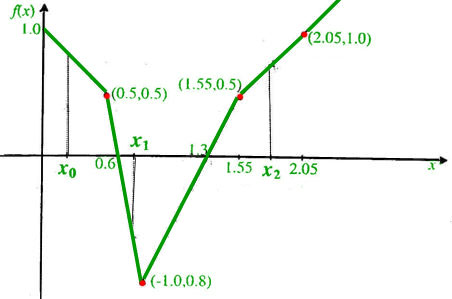Open in App
Not now

# GATE | GATE-CS-2003 | Question 42

• Last Updated : 14 Feb, 2018

A piecewise linear function f(x) is plotted using thick solid lines in the figure below (the plot is drawn to scale).If we use the Newton-Raphson method to find the roots of f(x) = 0 using x0, x1 and x2 respectively as initial guesses, the roots obtained would be
(A) 1.3, 0.6, and 0.6 respectively
(B) 0.6, 0.6, and 1.3 respectively
(C) 1.3, 1.3, and 0.6 respectively
(D) 1.3, 0.6, and 1.3 respectively

Explanation: First of all, There is a mistake in coordinates of a given point. I have corrected that in red color.Now in Newton-Raphson method, we draw a tangent from our guess point, and our new guess would be the point where this tangent cuts x-axis. Now we choose initial guess points one by one :

```
x0 : Tangent at this point is line AB itself, and that would cut x-axis at
point (1.0,0.0)  (found using equation of line AB). So our next guess
would be 1.0. Point on the curve corresponding to this new guess 1.0
is shown as F. Now tangent at point F is line DE,   which cuts x-axis at
1.3, and at this point, value of function is zero, so we found  the root
as 1.3.

x1 : Tangent at this point is line BE, which cuts x-axis at 0.6, also function
value is zero here, so we find root as 0.6.

x2 : Tangent at this point is line CD, which cuts x-axis at 1.05 (again found
by finding equation of line CD). Point on the curve corresponding to this
new guess 1.05 is shown as G. Now tangent at point G is line DE, which cuts
x-axis at 1.3, and at this point, value of function is zero, so we found
the root as 1.3. ```

Source: Question 60 of http://www.cse.iitd.ac.in/~mittal/gate/gate_math_2003.html

My Personal Notes arrow_drop_up
Related Articles